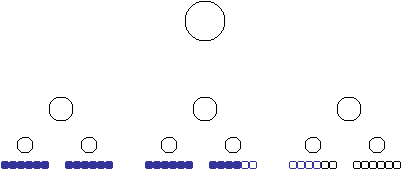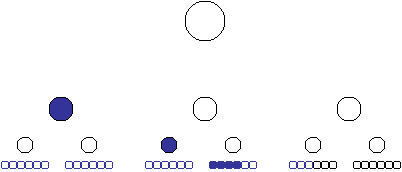# Inventory Management Calculations in SQL Server Analysis Services 2005

A common business problem is how to calculate inventory based on incoming and outgoing counts. At any point in time, inventory is not simply summed from the deltas because inventory on a particular day is the sum of the deltas across time. Additionally, inventory is counted on a regular basis to account for errors in deltas, shrinkage, or other discrepancies.

For example, consider a warehouse containing widgets and sprockets. As widgets and sprockets are shipped in, a positive increment is recorded. As they are shipped out, a negative increment is recorded.  Once in a while, a snapshot count is made as a new baseline from which future increments are added and subtracted. The number of widgets and sprockets in the warehouse at any point in time is the sum of the most recent snapshot (or tally) plus the positive and negative increments that happened after that snapshot.

This document explains how these problems can be solved with MDX Scripting in Analysis Services 2005. The reader is assumed to possess moderate to advanced mdx skills.

Special Note: This is not complete as usage of semi-additive measures is not included. Stay tuned for updates.

## Cube Structure

The cube has the following dimensions:

Warehouse
Product
Time

The calculations below depend on the Warehouse and time dimension only. Other dimensions can be added without affecting the expressions or their performance.

The following measures are referred to in this document:

 Value Source Description Units Delta Fact table The change in the count of a product in a warehouse at a certain point in time. This comes from the fact table. Running Delta Sum Calculated Measure The running sum from the beginning of time. This is a working calculated member and would not be exposed. Units Tallied Fact Table Snapshot inventory counts. The snapshot count is assumed to take place on the same day for all products in each warehouse (but counts can be taken in different warehouses on different days). Deltas Up To Last Tally Calculated Measure The Sum of the incremental changes from the first point in time to the current point. This is a working calculation and would not be exposed. Units Last Tally Fact Table* The amount of the most recent snapshot inventory count. This is an interim calculation and would not be exposed. Units Net Fact Table* The inventory count at any point in time

*Note that these values are populated by assignments described below. The measures are sourced from the fact table because calculated measures do not aggregate and measures sourced from the fact table do.

## Summing Increments

For any given warehouse and product, the running delta sum is the sum of Units Delta from the beginning of time. There is an important thing in this statement: the algorithm is the same for any product at any warehouse. Therefore, the calculation doesn’t have to be made for each individual warehouse or product and the results summed up a hierarchy (this would hurt performance).

A common way to sum a measure from the beginning of time looks like this:

Create Member Measures.[Running Delta Sum] as
sum(
[Time].[Calendar].[Date].members(0):
ClosingPeriod([Time].[Calendar].[Date]),
Measures.[Units Delta]
)
, visible=false;

This expression sum [Units Delta] from the first member to the last descendant at the date level of the currentmember in Time (so querying a year returns the inventory as of the last day in the year).

This works, but it can be made more performant. Analysis Service excels at calculating aggregates. Instead of summing values across days, aggregate values can be used.

Consider the diagram below:Determining the sum of delta for any point in time is the sum of the prior days – colored in blue. But this sum can be calculated differently:In other words, the sum of deltas at any point in time is the sum of the member plus its prior siblings plus its parent’s prior siblings, its parent’s parent’s prior siblings and so on.

In mdx this is:

Create Member Measures.[Running Delta Sum] as
sum(
generate
(
ascendants([Time].[Calendar].currentmember),
iif(
[Time].[Calendar].currentmember IS
[Time].[Calendar].firstsibling,
{},
[Time].[Calendar].firstsibling:
[Time].[Calendar].prevmember
)
) + [Time].[Calendar].currentmember
,
[Measures].[Units Delta]
)
, visible=false;

This may take some explaining:

-         The sum function sums the Units Delta measure
-         The generate function applies the set expression to each ascendant member of the current member in time.
-         There is a check to see if the member is the first member among its siblings because the sum includes prior siblings of ascendant members but not the ascendant member itself.

## Dealing with Snapshot Counts

Inventory management entails counting everything once in a while and then continuing the delta from that point. So the real calculation takes a look at the last snapshot and continues the sum from there.

The algorithm used here is to calculate the sum of the deltas from the first point in time up to the last snapshot inventory and subtract that from the sum of deltas from the same first point in time to the current point in time. The difference is the sum of deltas from the last snapshot. Adding that value to the snapshot gives us the current count.

The (hidden) calculated measure [Deltas up to Last Snapshot] has the expression :

Create member [Measures].[Deltas Up to Last Tally] as
sum(
generate(
ascendants(
{tail(
nonemptycrossjoin(
[Time].[Calendar].[Date].members(0):
ClosingPeriod([Time].[Calendar].[Date]),
{[Measures].[Units Tallied]},
1)
,1)+
// The union with the first member is to cover no snapshots.
[Time].[Calendar].[Date].members(0)}.item(0)
),
iif(
[Time].[Calendar].currentmember IS
[Time].[Calendar].firstsibling,
{},
[Time].[Calendar].firstsibling:
[Time].[Calendar].prevmember
)
)
,[Measures].[Units Delta]
)
, visible=false;

This expression is a simple extension of the algorithm used earlier. The only difference is this set in the middle:

nonemptycrossjoin(
[Time].[Calendar].[Date].members(0):
ClosingPeriod([Time].[Calendar].[Date]),
{[Measures].[Units Tallied]},
1)
,1)

This is the point in time when the last snapshot count was made.

## Sidebar: NonEmptyCrossJoin or Recursion

(This is an advanced topic that can be skipped.)

There are two commonly used methods for getting the last non empty member at a level. The more common, and usually more performant, method is using NonEmptyCrossJoin function as described above. But there is another: recursion.

Consider the diagram below:Given any starting point, we want to determine the last non empty value. A calculated member can check to see if its last descendant is empty and return the value if its not, or call itself on the prior member if it is. Like this:

with member measures.[LastNonEmptyValue] as
iif(
IsEmpty( (Measures.[Value],
ClosingPeriod([Time].[Calendar].[Date]) ) )
,
(measures.[LastNonEmptyValue],
ClosingPeriod([Time].[Calendar].[Date]).PrevMember)
,
Measures.[Value]
)

Starting at any point in the hierarchy, the navigation of this recursive calculated member goes like this to find the last non empty value:And this is just fine for small hierarchies or when most members are non empty. But consider what happens when the number of members at the lowest level gets large – the calculated member is evaluated again and again until that member with a value is found.

So reconsider the algorithm. Assuming the hierarchy is additive, we know ascendant member will be non empty if any of its descendants are non empty.  So the number of calculations can be reduced if the recursion goes up in time until it finds a value and then it goes down in time until it finds a leaf member containing that last value. Returning to the diagram, this looks like:In mdx this is:

with member measures.[LastNonEmptyValue] as
iif(
IsEmpty( Measures.[Value] ),
iif(Time.parent.firstchild is Time.currentmember,
(Measures.[LastNonEmptyValue], Time.parent.prevmember),
(Measures.[ LastNonEmptyValue], Time.PrevMember)
)
,
iif(time.level IS [Time].[Calendar].[Date],
Measures.[Value],
(measures.[LastNonEmptyValue],time.lastchild)
)
)

One last thing – the expression must be protected against the condition when there is no prior non empty member. This is done by encasing the expression with one more IIF:

iif(time.currentmember is null,
null
,
iif(
IsEmpty( Measures.[Value] ),
iif(Time.parent.firstchild is Time.currentmember,
(Measures.[LastNonEmptyValue], Time.parent.prevmember),
(Measures.[ LastNonEmptyValue], Time.PrevMember)
)
,
iif(time.level IS [Time].[Calendar].[Date],
Measures.[Value],
(measures.[LastNonEmptyValue],time.lastchild)
)
)
)

So returning to the inventory problem, we have a choice to use one of these recursive expressions or the NonEmptyCrossJoin. Which one?

There isn’t a simple answer. Most of the time the NonEmptyCrossJoin function is better. But if there are frequent updates on large dimension, this recursive approach may prove superior. Test both.

One more thing to consider is complexity. Recursion is elegant, but difficult to comprehend. Even if you get a slight performance boost, consider the individuals maintaining your application.

## Wrap-up

Now we’re ready to make the final calculations:

scope(leaves([Warehouse]));
/*
Copy forward the most recent tally on any given day, month, quarter or year.
*/
[Measures].[Units Last Tally] =
tail(
// Take the first member in case the necj returns an empty set.
{([Time].[Calendar].[Date].members(0),[Measures].[Units Tallied])} +
//This returns the last day where with data.
NONEMPTYCROSSJOIN(
[Time].[Calendar].[Date].members(0):
ClosingPeriod([Time].[Calendar].[Date]),
{[Measures].[Units Tallied]}
)
,
1
).item(0);

/*
Calculate net units as the total of the running delta sum minus the sum of the deltas to the last time a tally was taken plus the tally.
*/
[Measures].[Units Net] =
[Measures].[Running Delta Sum] –
[Measures].[Deltas Up to Last Tally] +
[Measures].[Units Last Tally];
end scope;

Note that the scope is on the leaves of the warehouse dimension. This is done because individual warehouses take inventory at different points in time.  The final inventory calculation is done per warehouse and the sum of products across warehouses is the sum of the inventory calculations.

As the comments indicate, the first expression in the scope copies forward the last tally using the same Tail(…NonEmptyCrossJoin(… approach described earlier.

And the last assignment simply subtracts the sum of increments up to the last tally from the sum of all increments and adds to it the value of the last tally.

Tags: design, inventory

2007-2015 VidasSoft Systems Inc.